Chapter 16

### As we know the atom consisted of electrons and nucleus, and the nucleus consists of protons and neutrons.

Both protons and neutrons have the half spin just like electrons do. But the proton has one positive electric charge unit ‘e’ and the neutron as a whole has zero electric charge.

Comparing protons and electrons, the proton’s mass is much larger than the electron’s mass, but they have the same amount of electric charge (with different signs), protons have positive electric charges, and the electrons have negative electric charges, both the electrons and protons have the same value of half spin.

Let us make an assumption that the proton has a similar internal structure as electrons, the difference is that the proton has one positive electric charge, and the mass of the proton is much larger than that of the electron.

The electric charge density distribution inside the proton is as follows: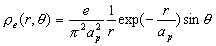(16.1)

The magnetic charge density distribution inside the proton is as follows: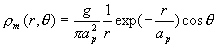(16.2)

Based on the above charge distribution equation, we can get the proton’s electromagnetic field distribution equation as follows: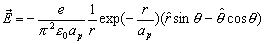(16.3)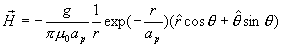(16.4)

Based on the electromagnetic field distribution equation, similar to the electron, we can get the proton’s electromagnetic field energy: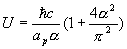(16.5)

Then, we make the assumption similar to the electron that the proton mass also has electromagnetic origin, then we have: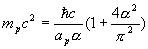(16.6)

Compare this with the electron energy formula: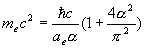(16.7)

So we can get following relationship:

###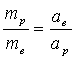(16.8)

As we know that: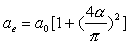(16.9)

Thus: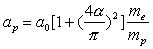(16.10)

In within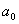is the Bohr radius.